# Born Haber Cycle Questions

The Born Haber process, also known as the Born Haber cycle, is a method for observing and analysing energies in a reaction. It primarily aids in the description of the formation of ionic compounds from various elements. The methodology also allows us to comprehend the overall reaction process via a series of steps.

The Born-Haber cycle was developed in 1919 by German scientists Fritz Haber and Max Born. The Born Haber cycle is primarily used to compute lattice energy. It also includes several steps or processes such as electron affinity, ionisation energy, sublimation energy, formation heat, and dissociation energy.

Definition: Born Haber cycle is a cycle of enthalpy change of process that leads to the formation of a solid crystalline ionic compound from the elemental atoms in their standard state and of the enthalpy of formation of the solid compound such that the net enthalpy becomes zero.

## Born Haber Cycle Chemistry Questions with Solutions

Q1. Which of the following can be calculated from the Born-Haber cycle for Al2O3?

a.) The lattice energy of Al2O3

b.) Electron affinity of O-atom

c.) The ionization energy of Al

d.) All of these

Correct Answer- (d.) All of these

Q2. The Born Haber cycle below represents the energy changes occurring at 298K when KH is formed from its elements

v : ΔHatomisation K = 90 kJ/mol

w : ΔHionisation K = 418 kJ/mol

x : ΔHdissociation H = 436 kJ/mol

y : ΔHelectronaffinity H = 78 kJ/mol

z : ΔHlattice KH = 710 kJ/mol

In terms of the letters v to z the expression for

ΔHi of K is ΔHi = w/2.

If true enter 1, else enter 0.

a.) 0

b.) 1

c.) 2

d.) 3

Q3. The Born Haber cycle below represents the energy changes occurring at 298K when KH is formed from its elements

v : ΔHatomisation K = 90 kJ/mol

w : ΔHionisation K = 418 kJ/mol

x : ΔHdissociation H = 436 kJ/mol

y : ΔHelectronaffinity H = 78 kJ/mol

z : ΔHlattice KH = 710 kJ/mol

On complete reaction with water, 0.1g of KH gave a solution requiring 25 cm3 of 0.1M HCl for neutralization. Calculate the relative atomic mass of potassium from this information.

a.) 39

b.) 40

c.) 41

d.) 42

Q4. The Born Haber cycle below represents the energy changes occurring at 298K when KH is formed from its elements

v : ΔHatomisation K = 90 kJ/mol

w : ΔHionisation K = 418 kJ/mol

x : ΔHdissociation H = 436 kJ/mol

y : ΔHelectronaffinity H = 78 kJ/mol

z : ΔHlattice KH = 710 kJ/mol

Choose the correct value of ΔH.

a.) 124 KJ/mol

b.) -124 KJ/mol

c.) 12 J/mol

d.) None of these

Correct Answer – (b.) -124 KJ/mol

Q5. The Born Haber cycle below represents the energy changes occurring at 298K when KH is formed from its elements

v : ΔHatomisation K = 90 kJ/mol

w : ΔHionisation K = 418 kJ/mol

x : ΔHdissociation H = 436 kJ/mol

y : ΔHelectronaffinity H = 78 kJ/mol

z : ΔHlattice KH = 710 kJ/mol

In terms of the letters v to z the expression for ΔHelectronaffinity of H is-

a.) y

b.) y/2

c.) 2y

d.) y/3

Q6. Write some examples of the Born Haber Cycle?

• A solid magnesium atom sublimes to a gaseous atom by absorbing heat energy (Hsub).
• Magnesium atoms in gaseous form emit two electrons with matching ionization energies in two stages.

Q7. The following data relate to lithium chloride. The standard molar enthalpy change of solution is -37.0 kJ mol–1. Lattice enthalpy is -846 kJ mol–1. Give the name of each of the changes A and B.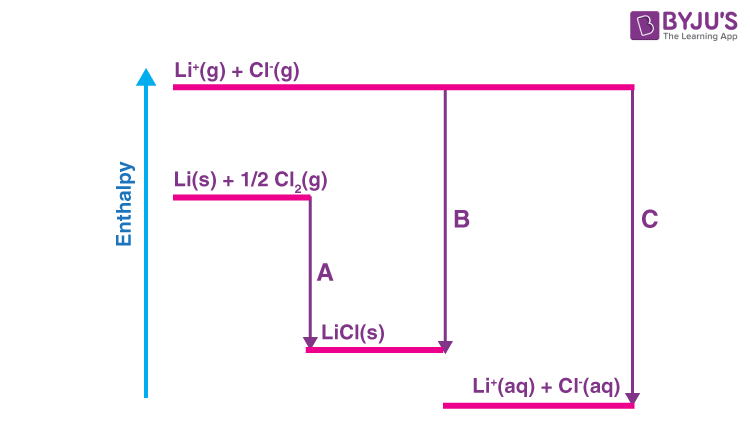Answer. A is (enthalpy change) of formation and B is lattice enthalpy

Q8. Calculate the value of the enthalpy change represented by C and suggest the name(s) of the enthalpy change(s).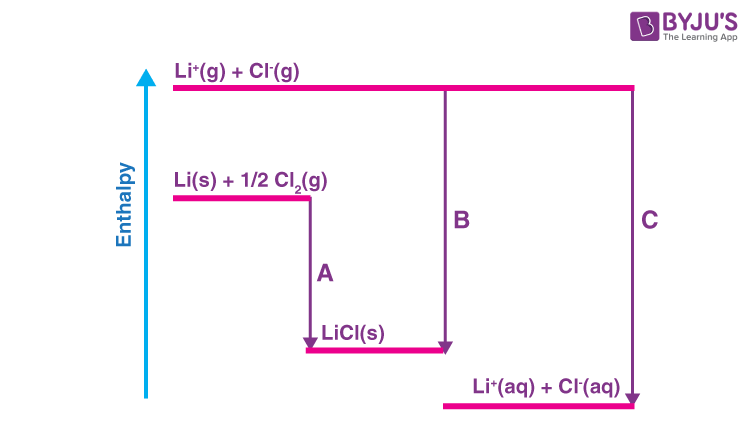Answer. – 846+ – 37 = – 883 kJ mol–1

Hydration/Solvation enthalpies of Li+ and Cl.

Q9. What distinguishes Hess law from the Born Haber cycle?

Answer. Hess’s Law states that the overall change in energy of a process can be calculated by breaking it down into parts and then adding the energy changes of each phase. In the Born-Haber Cycle, Hess’ Law is effectively applied to an ionic solid. The Born Haber cycle is employed to calculate electron affinity, crystal energy, and lattice energy.

Q10. The energy level diagram (Born-Haber cycle) for caesium chloride is shown below. Give the names of the enthalpy changes represented by ∆H1, ∆H2, and ∆H5.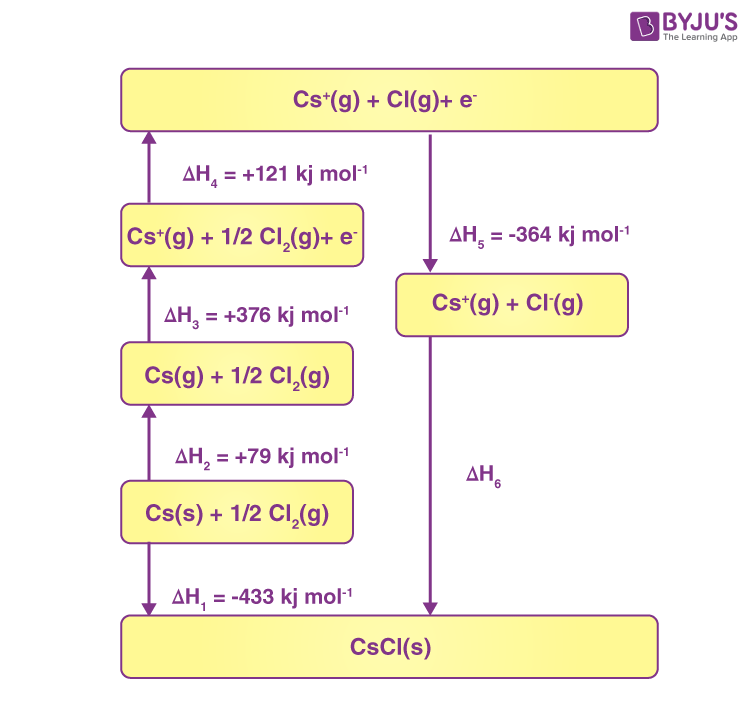∆H2 – atomization/sublimation of Cs

∆H5 – electron affinity of Cl

Q11. The energy level diagram (Born-Haber cycle) for caesium chloride is shown below. Calculate the value of the lattice energy ∆H6.Answer. -433 = 79 + 376 + 121 – 364 + ∆H6

∆H6 = -645 (kJ mol–1)

Q12. The energy level diagram (Born-Haber cycle) for caesium chloride is shown below. Explain why the enthalpy change represented by ∆H3 has a lower magnitude for caesium than for sodium.Answer. Cs has larger radius / larger atom (not ion) / more shells / more orbitals / more sub-shells. So more shielding

So, less powerful attraction (of the nucleus) for (outer) e in Cs

Q13. Figure 1 shows the energy level diagram (Born-Haber cycle) for forming rubidium iodide from its elements. Complete the diagram giving the identities of the missing species.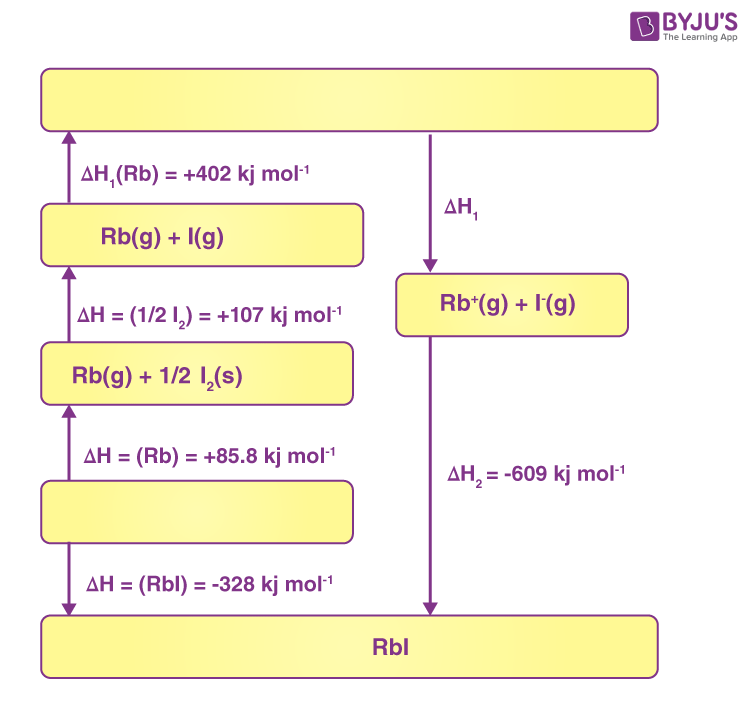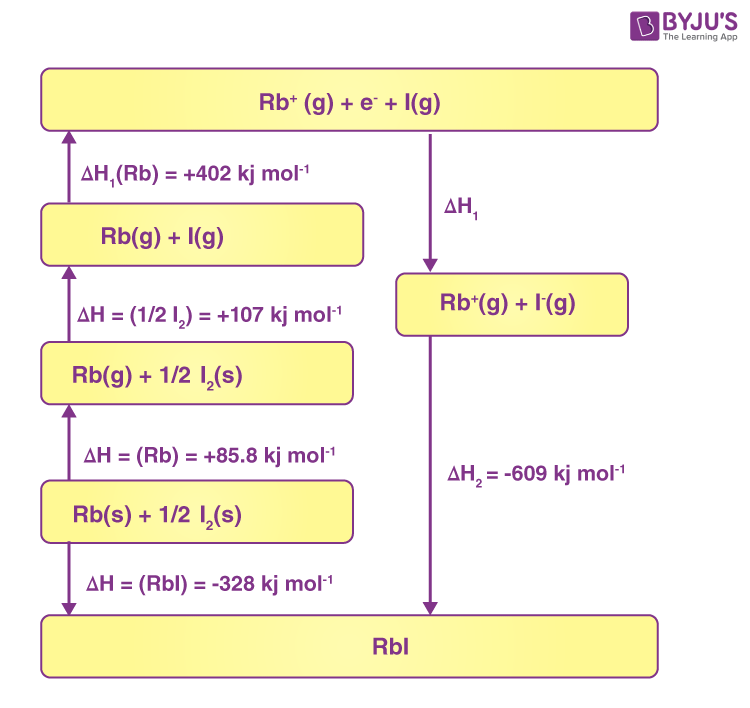Q14. Give the names of the enthalpy changes represented by ∆H1 and ∆H2.

Answer. ∆H1 – electron affinity of iodine

∆H2 – lattice enthalpy of RbI

Q15. Calculate the value of the enthalpy change represented by ∆H1.

Answer. ∆H1 = -(+402) – (+107) – (+85.8) + (-328) – (-609)

= -314 (kJ mol–1)

## Practise Questions on Born Haber Cycle

Q1. Born Haber Cycle is used to determine-

a.) Lattice energy

b.) electron affinity

c.) ionization energy

d.) either of them

Q2. What type of enthalpy cycle is used to find out the solution for lattice energy?

a.) Hess’s Law

b.) Born-Haber cycle

c.) Haber process

d.) Contact process

Q3. How does the Born-Haber cycle explain the stability of ionic compounds?

Q4. What is the limitation of the Born-Haber cycle?

Q5. How to Apply the Born-Haber Cycle

Click the PDF to check the answers for Practice Questions.